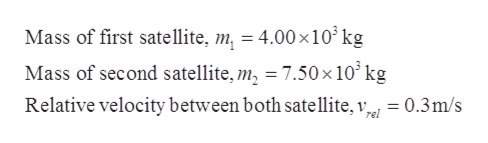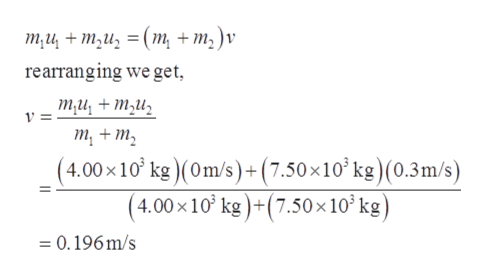# Two manned satellites approaching one another at a relative speed of 0.300 m/s intend to dock. The first has a mass of 4.00 ✕ 103 kg, and the second a mass of 7.50 ✕ 103 kg. Assume that the positive direction is directed from the second satellite towards the first satellite.(a) Calculate the final velocity after docking, in the frame of reference in which the first satellite was originally at rest. ..............m/s

Question

Two manned satellites approaching one another at a relative speed of 0.300 m/s intend to dock. The first has a mass of 4.00 ✕ 103 kg, and the second a mass of 7.50 ✕ 103 kg. Assume that the positive direction is directed from the second satellite towards the first satellite.

(a) Calculate the final velocity after docking, in the frame of reference in which the first satellite was originally at rest.
..............m/s

check_circleExpert Solution
Step 1

Given,help_outlineImage TranscriptioncloseMass of first satellite, m = 4.00x10 kg Mass of second satellite, m2 7.50 x 10° kg Relative velocity between both satellite, v 0.3m/s fullscreen
Step 2

In the frame of the first satellite, if we assume the direction of the approaching velocity of second satellite as positive.

Then, the velocity of both satellites are,

Step 3

After docking both satellite attains equal velocity v.

Applying the conservation of momentu...help_outlineImage Transcriptioncloseти + т,и, 3D (т, + т,)v rearranging we get ти, + ти, у 3D т, + т, (4.00х10' kg)(Om/s)+ (7.50x10* k?) (0.3m/s) (4.00х10' ke)+(7.50 x10'kg) =0.196m/s fullscreen

### Want to see the full answer?

See Solution

#### Want to see this answer and more?

Solutions are written by subject experts who are available 24/7. Questions are typically answered within 1 hour*

See Solution
*Response times may vary by subject and question
Tagged in

### Collisions